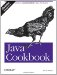# Recipe 5.4 Taking a Fraction of an Integer Without Using Floating Point

#### Problem

You want to multiply an integer by a fraction without converting the fraction to a floating-point number.

#### Solution

Multiply the integer by the numerator and divide by the denominator.

This technique should be used only when efficiency is more important than clarity, as it tends to detract from the readability and therefore the maintainability of your code.

#### Discussion

Since integers and floating-point numbers are stored differently, it may sometimes be desirable and feasible, for efficiency purposes, to multiply an integer by a fractional value without converting the values to floating point and back, and without requiring a "cast":

`/** Compute the value of 2/3 of 5 */ public class FractMult {     public static void main(String u[]) {         double d1 = 0.666 * 5;    // fast but obscure and inaccurate: convert         System.out.println(d1); // 2/3 to 0.666 in programmer's head         double d2 = 2/3 * 5;    // wrong answer - 2/3 == 0, 0*5 = 0         System.out.println(d2);         double d3 = 2d/3d * 5;    // "normal"         System.out.println(d3);         double d4 = (2*5)/3d;    // one step done as integers, almost same answer         System.out.println(d4);         int i5 = 2*5/3;            // fast, approximate integer answer         System.out.println(i5);     } }`

Running it looks like this:

`\$ java  FractMult 3.33 0.0 3.333333333333333 3.3333333333333335 3 \$`Java Cookbook, Second Edition
ISBN: 0596007019
EAN: 2147483647
Year: 2003
Pages: 409
Authors: Ian F Darwin

Similar book on Amazon